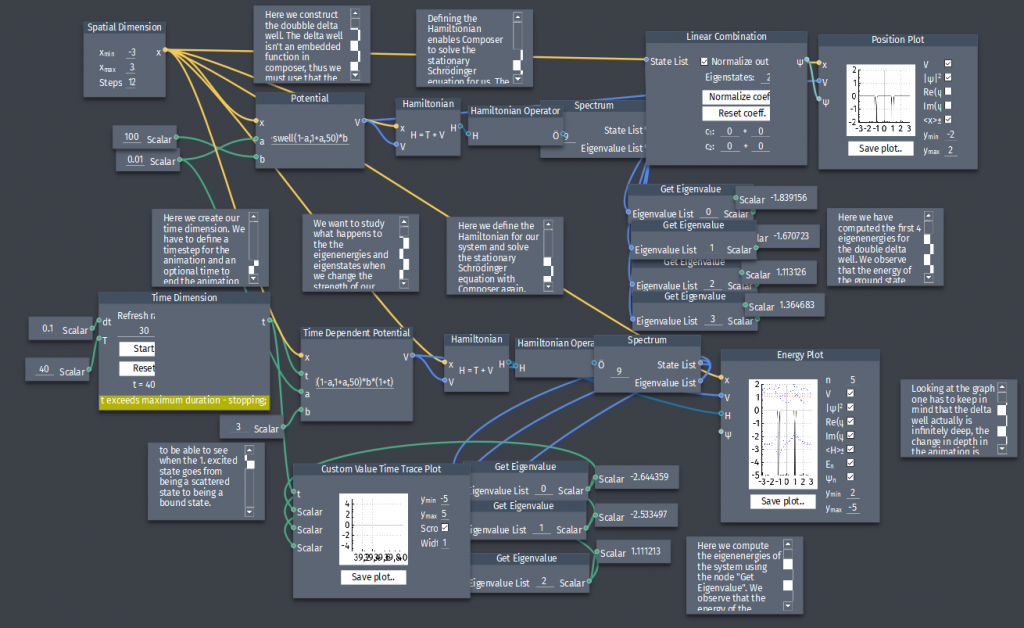# Double delta-function potential

Consider the double delta-function potential

$V(x)=-\alpha\left[\delta(x+a)+\delta(x-a)\right]$

where $\alpha$ and $a$ are positive constants.

a) Plot the potential.

b) Compute the eigenenergies for the ground state and the first 3 excited states.

c) Let the depth of the potential develop in time, plot and observe the evolution of the eigenenergies.